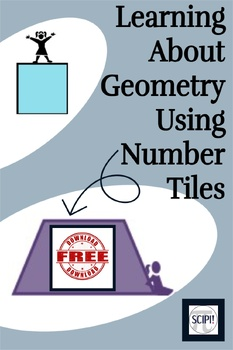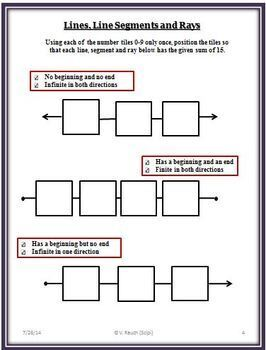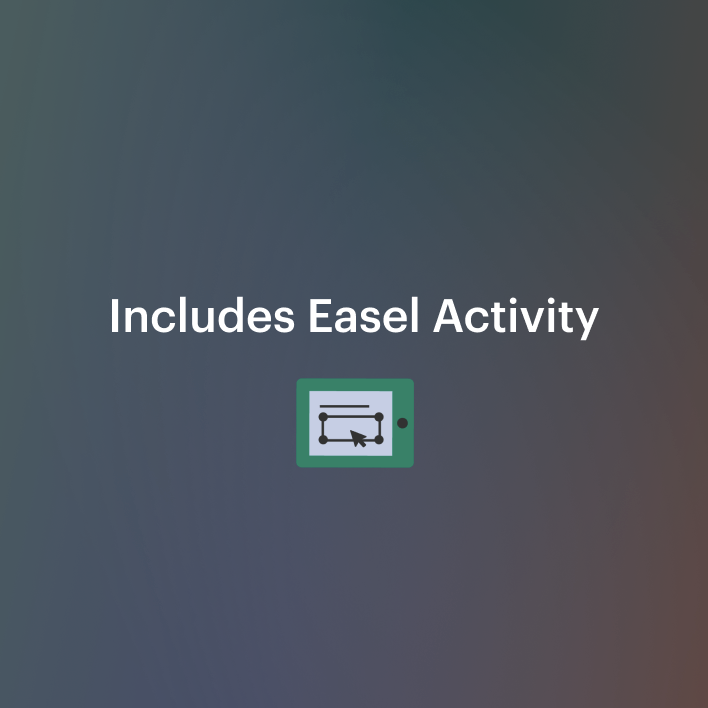# FREE Math Geometric Puzzles - Learning About Plane Geometry Using Number Tiles5th - 8th, Homeschool
Subjects
Resource Type
Formats Included
• PDF
•Activity
Pages
9 pages
Easel Activity Included
This resource includes a ready-to-use interactive activity students can complete on any device. Easel by TpT is free to use! Learn more.

### Description

All of the pages of this FREE math geometric puzzle resource are solved like magic squares. Number tiles are positioned so that the total sum of the tiles on each line of the geometric shape must add up to be the same. The designated sum for the shape is written on each page as well as the specific number of tiles to be used.

Before each set of activities, the geometry vocabulary used for that group is listed. The definitions include diagrams and/or illustrations so that the students can learn and understand new math words without difficulty or cumbersome words.

Ten number tiles are required for each of these four puzzles. The students use each number tile only once to solve each activity; however, some of the activities will not require the use of all ten tiles; so, reading and following the directions before beginning each geometric puzzle is imperative. Most of the geometric puzzles have more than one answer; so, students are challenged to find a variety of answers.

An answer recording sheet is provided for the student as well as possible solutions for the teacher. A number tile blackline is also included. This resource is EASEL ready. All you have to do is assign the pages to your students.

If your students enjoy the challenge of using number tiles, you might also enjoy:

Number Tile Math Activities

The A, B, C's of Number Tiles

Number Tiles – Hands On Math Activities for the Primary Grades

Number Tiles Problem Solving Math Hands-On Activities, Making Magic Numbers

You can also purchase a Number Tile Math Bundle which will save you money:

Number Tiles Math Bundle – 67 Problem Solving/Critical Thinking Math Activities Using Number Tiles

Total Pages
9 pages
Included
Teaching Duration
N/A
Report this Resource to TpT
Reported resources will be reviewed by our team. Report this resource to let us know if this resource violates TpT’s content guidelines.